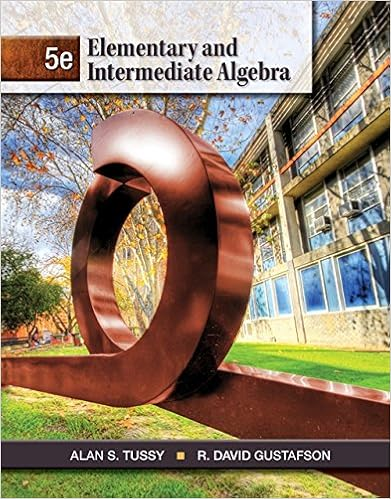# B a new path and a bridge are constructed from point

• 8

This preview shows page 8 out of 8 pages.

##### We have textbook solutions for you!
The document you are viewing contains questions related to this textbook.The document you are viewing contains questions related to this textbook.
Chapter 6 / Exercise 27
Elementary and Intermediate Algebra
Tussy/GustafsonExpert Verified
b. A new path and a bridge are constructed from point Q to the midpoint M of } PR . Find QM to the nearest yard. c. A man jogs from P to Q to M to R to Q and back to P at an average speed of 150 yards per minute. About how many minutes does it take? Explain . 40 20 0 X Y TAKS REASONING
54. CHALLENGE } AB bisects } CD at point M , } CD bisects } AB at point M , and AB 5 4 p CM . Describe the relationship between AM and CD . 1. Sketch two lines that intersect the same plane at two different points. The lines intersect each other at a point not in the plane. (p. 2) In the diagram of collinear points, AE 5 26, AD 5 15, and AB 5 BC 5 CD . Find the indicated length. (p. 9) 2. DE 3. AB 4. AC 5. BD 6. CE 7. BE 8. The endpoints of } RS are R ( 2 2, 2 1) and S (2, 3). Find the coordinates of the midpoint of } RS . Then find the distance between R and S . (p. 15) A B C D E Q UIZ for Lessons 1.1–1.3 EXTRA PRACTICE for Lesson 1.3, p. 896 ONLINE QUIZ at classzone.com 55. TAKS PRACTICE What are the solutions to x 2 1 4 x 5 5? TAKS Obj. 5 A x 5 1 and x 5 5 B x 5 1 and x 5 2 5 C x 5 2 1 and x 5 5 D x 5 2 1 and x 5 2 5 56. TAKS PRACTICE Juan is putting up a wallpaper border along the top of each wall in his rectangular living room. The border costs \$9.25 per roll plus 7.75% sales tax. One roll is 15 feet long. What other information is needed to determine the number of rolls of border he needs to purchase? TAKS Obj. 10 F The perimeter of the room G The total cost of each roll of border H The weight of one roll of border J The height of the walls in the room 57. TAKS PRACTICE Sally can jog at a rate of 6.5 miles per hour. If she continued in a straight path at this rate, what distance would she travel in 24 minutes? TAKS Obj. 9 A 2.2 miles B 2.4 miles C 2.6 miles D 2.8 miles M IXED R EVIEW FOR TAKS TAKS PRACTICE at classzone.com REVIEW Skills Review Handbook p. 882; TAKS Workbook REVIEW Skills Review Handbook p. 894; TAKS Workbook REVIEW Skills Review Handbook p. 894; TAKS Workbook
##### We have textbook solutions for you!
The document you are viewing contains questions related to this textbook.The document you are viewing contains questions related to this textbook.
Chapter 6 / Exercise 27
Elementary and Intermediate Algebra
Tussy/GustafsonExpert Verified
•••